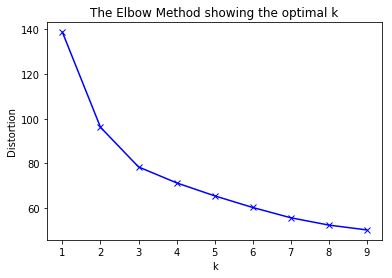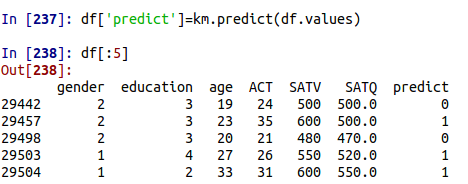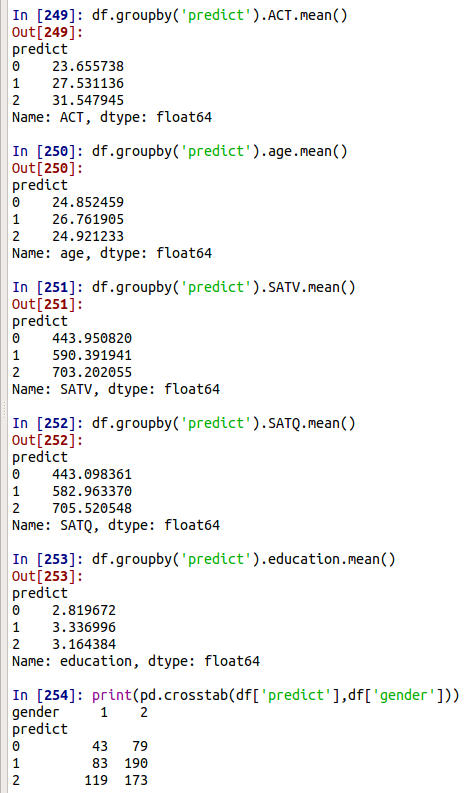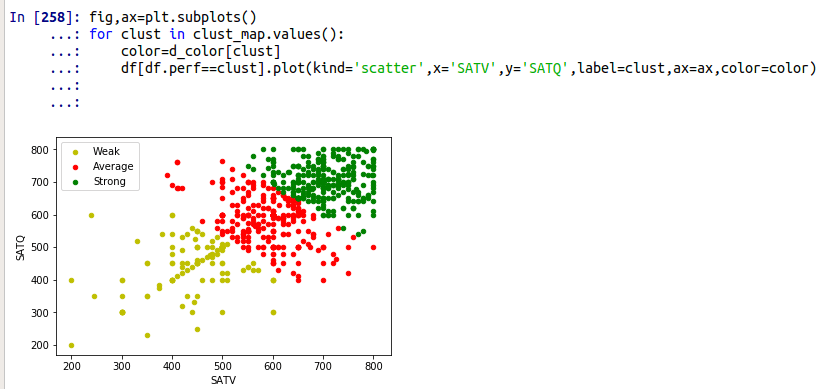# KMeans Clustering in Python

Kmeans clustering is a technique in which the examples in a dataset our divided through segmentation. The segmentation has to do with complex statistical analysis in which examples within a group are more similar the examples outside of a group.The application of this is that it provides the analysis with various groups that have similar characteristics which can be used to cater services to in various industries such as business or education. In this post, we will look at how to do this using Python. We will use the steps below to complete this process.

1. Data preparation
2. Determine the number of clusters
3. Conduct analysis

Data Preparation

Our data for this examples comes from the sat.act dataset available in the pydataset module. Below is some initial code.

```import pandas as pd
from pydataset import data
from sklearn.cluster import KMeans
from scipy.spatial.distance import cdist
import numpy as np
import matplotlib.pyplot as plt```

We will now load our dataset and drop any NAs they may be presentYou can see there are six variables that will be used for the clustering. Next, we will turn to determining the number of clusters.

Determine the Number of Clusters

Before you can actually do a kmeans analysis you must specify the number of clusters. This can be tricky as there is no single way to determine this. For our purposes, we will use the elbow method.

The elbow method measures the within sum of error in each cluster. As the number of clusters increases this error decreases. However,  a certain point the return on increasing clustering becomes minimal and this is known as the elbow. Below is the code to calculate this.

```distortions = []
K = range(1,10)
for k in K:
kmeanModel = KMeans(n_clusters=k).fit(df)
distortions.append(sum(np.min(cdist(df, kmeanModel.cluster_centers_, 'euclidean'), axis=1)) / df.shape)```

Here is what we did

1. We made an empty list called ‘distortions’ we will save our results there.
2. In the second line, we told R the range of clusters we want to consider. Simply, we want to consider anywhere from 1 to 10 clusters.
3. Line 3 and 4, we use a for loop to calculate the number of clusters when fitting it to the df object.
4. In Line 5, we save the sum of the cluster distance in the distortions list.

Below is a visual to determine the number of clusters

```plt.plot(K, distortions, 'bx-')
plt.xlabel('k')
plt.ylabel('Distortion')
plt.title('The Elbow Method showing the optimal k')```The graph indicates that 3 clusters are sufficient for this dataset. We can now perform the actual kmeans clustering.

KMeans Analysis

The code for the kmeans analysis is as follows

```km=KMeans(3,init='k-means++',random_state=3425)
km.fit(df.values)```
1. We use the KMeans function and tell Python the number of clusters, the type of, initialization, and we set the seed with the random_state argument. All this is saved in the object called km
2. The km object has the .fit function used on it with df.values

Next, we will predict with the predict function and look at the first few lines of the modified df with the .head() function.You can see we created a new variable called predict. This variable contains the kmeans algorithm prediction of which group each example belongs too. We then printed the first five values as an illustration. Below are the descriptive statistics for the three clusters that were produced for the variable in the dataset.It is clear that the clusters are mainly divided based on the performance on the various test used. In the last piece of code, gender is used. 1 represents male and 2 represents female.

We will now make a visual of the clusters using two dimensions. First, w e need to make a map of the clusters that is saved as a dictionary. Then we will create a new variable in which we take the numerical value of each cluster and convert it to a string in our cluster map dictionary.

```clust_map={0:'Weak',1:'Average',2:'Strong'}
df['perf']=df.predict.map(clust_map)```

Next, we make a different dictionary to color the points in our graph.

`d_color={'Weak':'y','Average':'r','Strong':'g'}`Here is what is happening in the code above.

1. We set the ax object to a value.
2. A for loop is used to go through every example in clust_map.values so that they are colored according the color
3. Lastly, a plot is called which lines up the perf and clust values for color.

The groups are clearly separated when looking at them in two dimensions.

Conclusion

Kmeans is a form of unsupervised learning in which there is no dependent variable which you can use to assess the accuracy of the classification or the reduction of error in regression. As such, it can be difficult to know how well the algorithm did with the data. Despite this, kmeans is commonly used in situations in which people are trying to understand the data rather than predict.